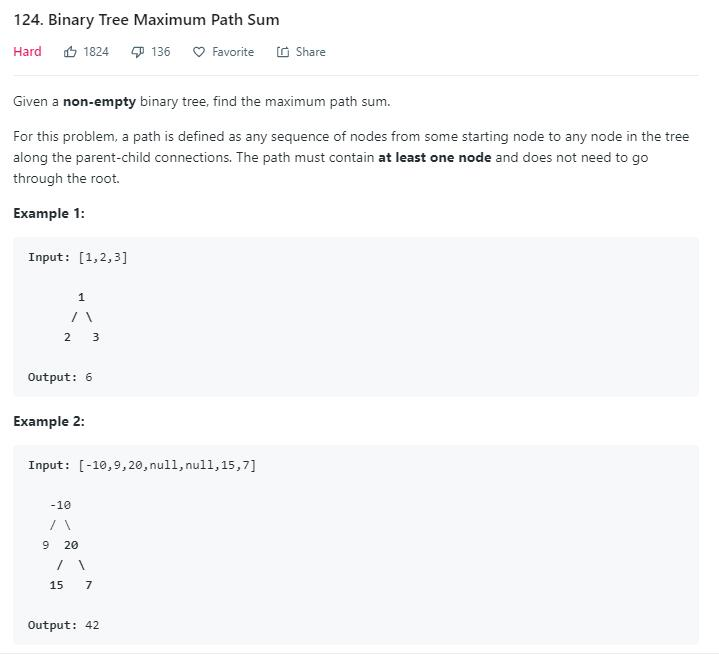# 题目描述（困难难度）# 解法一 递归

public int maxPathSum(TreeNode root) {
if (root == null) {
return Integer.MIN_VALUE;
}
//左子树的最大值
int left = maxPathSum(root.left);
//右子树的最大值
int right = maxPathSum(root.right);
//再考虑包含根节点的最大值
int  all = ....;
return Math.max(Math.max(left, right), all);
}


     8
/ \
-3   7
/  \
1    4


      8
/  \
-3   7
/    \
1      4
\    / \
3  2   6




public int maxPathSum(TreeNode root) {
//如果最大值是负数，我们选择不选
int left = Math.max(helper(root.left), 0);
int right = Math.max(helper(root.right), 0);
return root.val + left + right;
}

int helper(TreeNode root) {
if (root == null) return 0;
int left = Math.max(helper(root.left), 0);
int right = Math.max(helper(root.right), 0);
//选择左子树和右子树产生的值较大的一个
return root.val + Math.max(left, right);
}


int max = Integer.MIN_VALUE;

public int maxPathSum(TreeNode root) {
helper(root);
return max;
}
int helper(TreeNode root) {
if (root == null) return 0;

int left = Math.max(helper(root.left), 0);
int right = Math.max(helper(root.right), 0);

//求的过程中考虑包含当前根节点的最大路径
max = Math.max(max, root.val + left + right);

//只返回包含当前根节点和左子树或者右子树的路径
return root.val + Math.max(left, right);
}